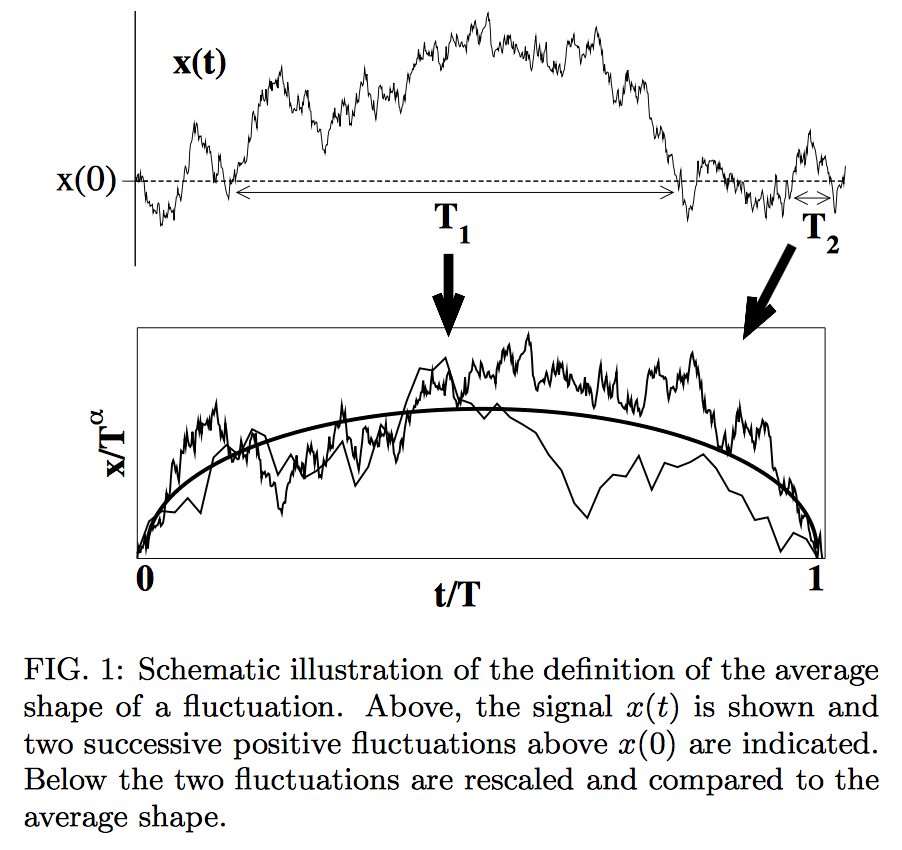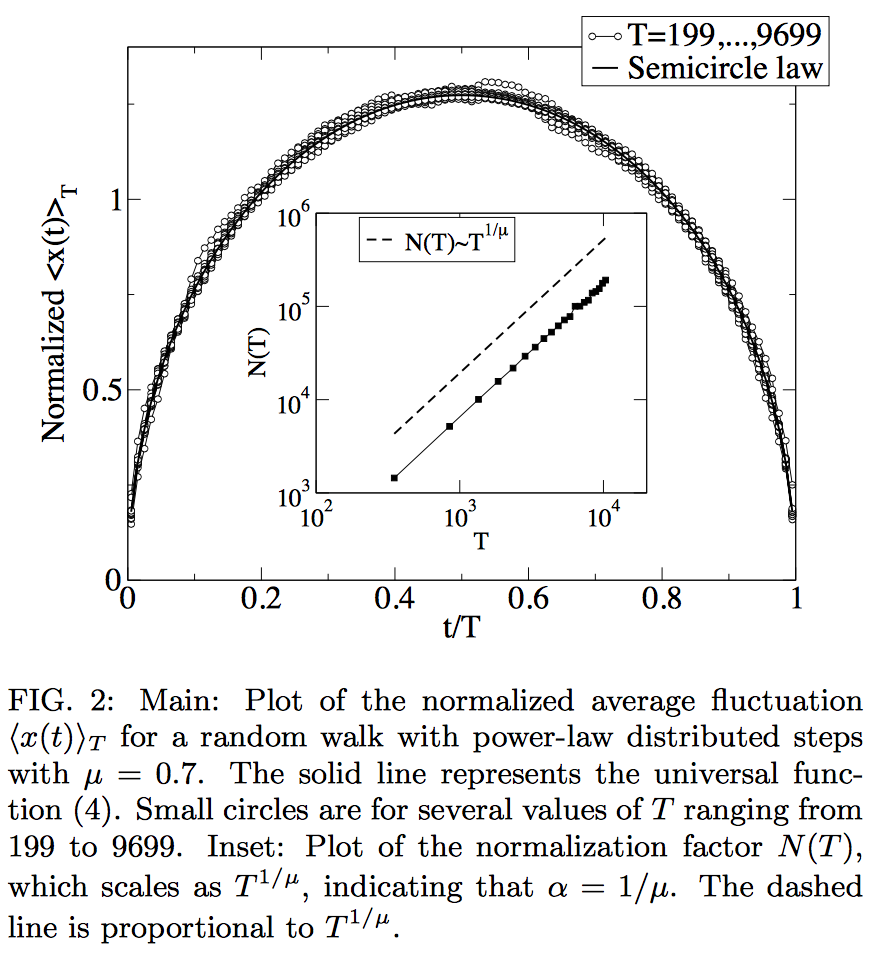# Semicircle law universality elsewhere

Wigner's semicircle distribution is:

$$f(x)=\frac{1}{2 \pi}\sqrt{4-x^2}, \ \ -2\leq x\leq 2.$$

Under reasonable conditions, the rescaled eigenvalue density of random symmetric matrices $M_n$ follows this distribution, from which we can infer both the number of rescaled eigenvalues in an interval $[a,b]$ and roughly where the $i$'th eigenvalue lies. Specifically, this concerns the statistics of the (unordered) vector $(\lambda_1(M),\lambda_2(M),\cdots,\lambda_n(M))$.

My question is, where else does the Wigner semicircle law arise? To push the discussion in a particular direction, I am primarily interested in other random processes $(X_1,\cdots,X_n)$ which asymptotically have semicircle statistics similar to the above. Specifically, I am not interested in processes which can be somehow coupled to random matrices. I would love to see some examples where the semicircle law occurs more or less universally, that is, for a wide, reasonable class of different distributions on $X_i$'s.

• I assume you are aware of Voiculescu's free probability theory? – Jon Bannon Jun 19 '15 at 22:52
• @jon bannon: I'm not intimately familiar with it but I basically lumped it with random matrix theory. If this is wrong of me I would love to see a note on this. – Alex R. Jun 19 '15 at 22:54
• The traces of $n$ independent random matrices in $SU(2)$? – Will Sawin Jun 19 '15 at 23:46
• @WillSawin The question seems to be asking for processes which asymptotically have distribution given by the (not-actually-semicircular) "semicircle law" rather than manifestations of the actual (not-actually-semicircular) semicircle law, which is as you observe the distribution of the trace of a random element of SU(2). Maybe the actual Sato-Tate conjecture would be the kind of thing the OP wants? – Yemon Choi Jun 20 '15 at 15:16

One broad area of research where the semicircle law appears is the study of complex networks (the internet, social networks of acquaintances, food webs, postal delivery routes,...). Quite generically, the degree distribution of the network follows a power law, meaning that the number of vertices of degree $j$ is proportional to $j^{−\beta}$ for some exponent $\beta$. The spectrum of the discrete Laplacian of such a power law graph follows the semicircle law if the minimum degree of a vertex is sufficiently large ($j_{\rm min}^2\gg j_{\rm average}$), see Spectra of random graphs with given expected degrees (Chung, Lu & Vu, 2003).

There are many more appearances of the Wigner distribution if you focus on the edge of the spectrum, rather than on the flat central region. Indeed, for applications to physics the semicircle itself is not particularly relevant (nor is it applicable), but it is the square-root singularity at the edge of the spectrum that matters. This includes the very first application by Eugene Wigner of the semicircle law to nuclear physics: there is not a single nuclear reaction where the resonances follow the semicircle law. In contrast, in typical physical systems that have a band gap (semiconductors, superconductors, ...), the density of states vanishes as a square root, in accordance with the semicircle law. Moreover, the fluctuations around the spectral edge have the same universal distribution as in Wigner's Gaussian ensemble --- even though the density of states away from the spectral edge is not semicircular.

• Is this semicircular law really different from the one OP was talking about? The adjacency matrix of a random graph is a random matrix; doesn't the Wigner law appear because this matrix is, well, similar enough to the GOE? – Kostya_I Jul 30 '17 at 21:50

Following up on the answers in the context of free probability theory one should note that there is actually a quite important and canonical operator on a Hilbert space having the semicircle as distribution. In the answers above there were already mentioned concrete operators arising from the free group representations, but there one also has to take the limit $d\to\infty$ to get the semicircle. I want to highlight a situation without taking a limit: Let $l$ be the one-sided shift (acting on a Hilbert space with orthonormal basis $(e_i)_{i\geq 0}$ via $le_i=e_{i+1}$), then the selfadjoint operator $l+l^*$ has with respect to the "vacuum expectation" $\langle e_0, \cdot e_0\rangle$ the semicirlce law as distribution. The one-sided shift is arguably one of the most important operators in single operator theory, but it seems nobody before Voiculescu was interested in looking at the distribution of its real part.

I learnt the following from Govind Menon.

Consider the Burgers equation $$\partial_t g+g \partial_x g=0$$ with initial condition $g(0,x)=1/x$. A self-similar solution is $g(t,x)=t^{-1/2}g_*(x\sqrt{t})$ and $g_*$ is obtained by analytic extension to $[-2,2]$ from the function $(z-\sqrt{z^2-4})/2$ which is the Stieltjes transform of the semicircle law!

(There is a bit of cheating going on here, as this appearance of Burgers equation can be deduced from taking limits involving Dyson's Brownian motion; still, at first sight it is surprising).

See http://www.dam.brown.edu/people/menon/publications/rm.pdf for more details.

Let me attempt another answer, more closely aligned to the request of the OP that the random process exhibiting the semicircle law should not be somehow coupled to random matrices. A random process of this type was studied in The average shape of a fluctuation: universality in excursions of stochastic processes (Baldassarri, Colairoir, and Castellano, 2003).

Consider the average shape of a fluctuation of a time series $$x(t)$$, that is the average value $$\langle x(t)\rangle_T$$ before $$x(t)$$ first returns, at time $$T$$, to its initial value $$x(0)=0$$. The random process is defined by $$x(t+1)=x(t)+\xi(t)$$ with independent steps $$\xi(t)$$ ($$t=0,1,2,3,\ldots$$). The cited reference finds the semicircle law $$\langle x(t)\rangle_T=T^{\alpha}f(t/T),\;\;f(s)=(8/\pi)^{1/2}\sqrt{s(1-s)}.$$ The exponent $$\alpha=1/2$$ if the steps have finite variance, because of some central limit theorem, but the semicircle law applies more generally: a Lévy distribution of the steps with heavy tails $$\propto 1/|\xi|^{1+\mu}$$ gives $$\alpha=1/\mu$$ with the same semicircle.

Here are two figures that illustrate the random process and the emerging semicircle. A quite remarkable universality.One-dimensional $\beta$-ensembles with quadratic potentials (Hermite/Gaussian ensembles) have the semi-circular law as equilibrium measure for any $\beta > 0$. It describes the large N behavior of a random process (the empirical measure on $N$ particles on the real line interacting via a pairwise logarithmic potential).

Of course for $\beta = 1,2,4$ you recover classical Random matrix models (well, for any $\beta$ there is a matrix model thanks to Dumitriu-Edelman), but they might be seen as systems of purely statistical physics nature, namely one-dimensional log-gases. If the potential is not quadratic then the semi-circular law does not appear necessarily, but what you get still looks a bit like it : the density vanishes like a square-root at the endpoints, see e.g. Kuijlaars-McLaughlin.

If you accept non-commutative random variables as "other random processes $(X_1,\ldots,X_n)$" then, as Jon Bannon's comment suggests, free probability could provide interesting examples [and I don't think it is fair to gather free probability and random matrix theory: If independent and (say, Wigner) random matrices are asymptotically free, it does not mean any free random variables comes from random matrix theory].

For instance, given any sequence $(X_n)$ of centered, normalized, self-adjoint, and free non-commutative random variables, the free central limit theorem states that the non-commutative moments of $$\frac{1}{\sqrt n}\sum_{i=1}^n X_i$$ convergs towards the moments of the semi-circle law.

Kerov shows (using his results on the asymptotics of Young diagrams) that the transition measure on Young diagrams has a semi-circle distribution (see Kerov's original paper here or this paper by Ivanov and Olshanski for a review). Similar results for the Plancherel measure have been obtained. One of the first results in this direction is the Baik-Deift-Johansson theorem that the longest increasing subsequence of random permutations (which by RSK is the first row of the Young diagram corresponding to that permutation) has the Tracy-Widom distribution (the distribution of the largest eigenvalue of random Hermitian matrices). The above paper by Ivanov and Olshanski extends that to the bulk.

• I would add the reference arxiv.org/pdf/1111.0575v4.pdf, which is related to Kerov's results. The semi-circle distributions appears there in several places, including for the position of a second-class particle in an interacting particle system that Romik-Sniady call Plancherel TASEP. – Guillaume Jun 30 '15 at 17:07

In the spirit of comments by Jon Bannon and Adrien Hardy, an example that precedes free probability theory but is part of it are random walk operators on free groups of increasing rank. More precisely, let $F(d)$ be the free group on $d$ generators, and consider the "Laplacian" for the random walk on the tree; its action on $\ell^2(F(d))$ is given by $\Delta_d = \frac{1}{2d} \sum_j \lambda(g_j)+\lambda(g_j^{-1})$, where $g_1,\dots,g_d$ are generators of the free group and $\lambda$ is the left-regular representation. The spectral measure of this operator was first computed by Kesten. I believe that he also observed that if one properly renormalizes the operator $\Delta_d$ (I think the normalization is $\sqrt{d}\Delta_d$) then in the limit $d\to\infty$ one obtains the semicircle law.

Of course, from the free probability standpoint this is just the free central limit theorem applied to operators $X_j = \lambda(g_j)+\lambda(g_j)^{-1}$ (each having arcsine law), which are freely independent almost by definition.